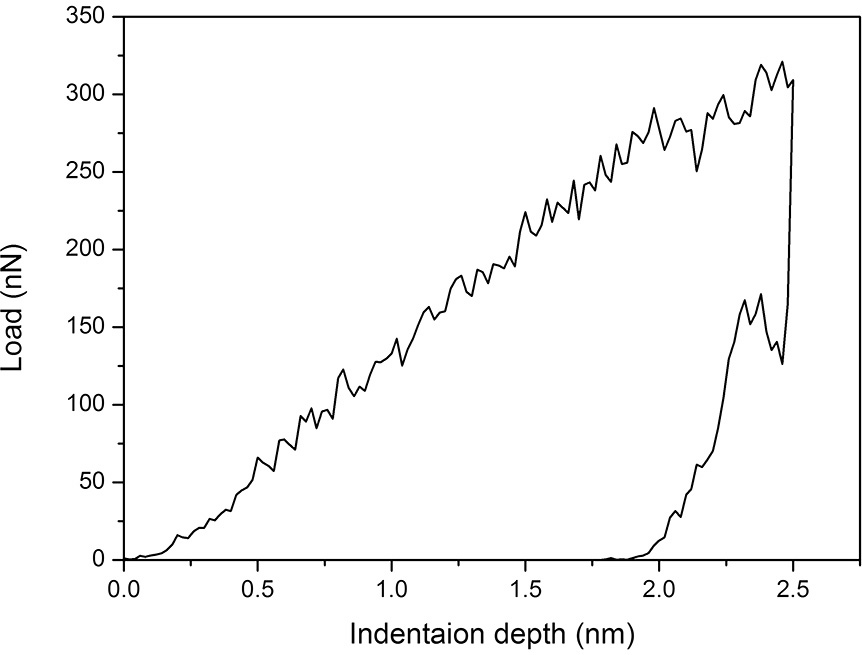# fix indent

Dear All,
I am using LAMMPS (14 Feb 2014) and have some questions about fix indent command:

1. The manual tells us that this fix computes a global scalar energy and a global 3-vector of forces (on the indenter). I wonder how LAMMPS calculates the global 3-vector of forces on the indenter (f_, f_ and f_) and whether they are related to the force of magnitude [F® = - K (r - R)^2] in the manual?

2. In some papers, we always see the load–displacement curves of the MD simulated samples during nanoindentation (just like the picture below or in the attachment). Is the scalar load (F) in the load–displacement curve obtained via the formula: F=sqrt(f_^2+ f_^2+f_^2)?
Here, f_, f_ and f_ are the global 3-vector of forces on the indenter.

1. The in.indent example script shows a fixed rate indentation. Because I want the indenter hold at the maximum indentation depth for 10000 steps before unloading (so-called hold stage), I add three lines of scripts in the end as below:

timestep 0.003
variable k equal 1000.0/xlat
variable y equal “13.0ylat - stepdt0.02ylat”

fix 4 all indent \$k sphere 10 v_y 0 5.0

run 30000
unfix 4

variable y1 equal “11.2*ylat”
fix 4 all indent \$k sphere 10 v_y1 0 5.0

run 10000

Am I right?

Thank you very much for your answer.
Best regards,The force on the indenter is conceptually simple. The indenter

imposes a force on some subset of atoms that are close enough

to it. The force on the indenter is simply the negative of the sum

of those per-atom forces.

Something like the code you list should hold the indenter at

one position and allow you to run in that mode for as long

as you like.

Steve